Anúncio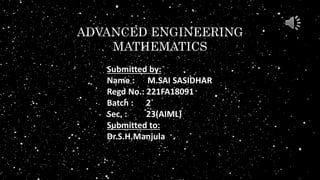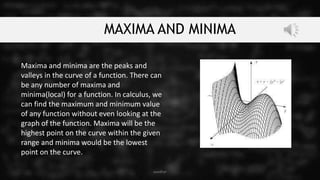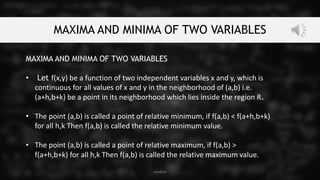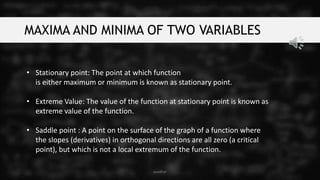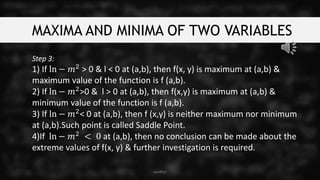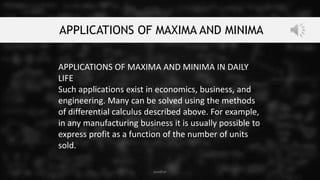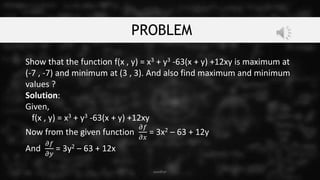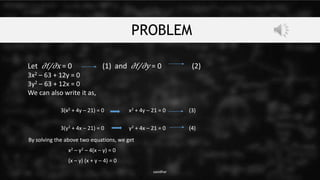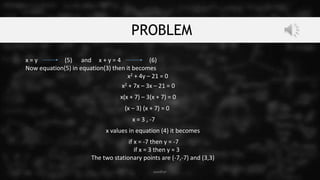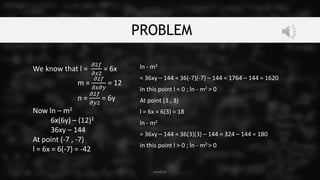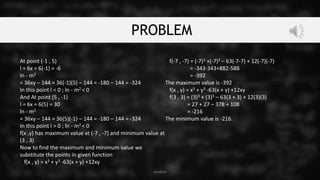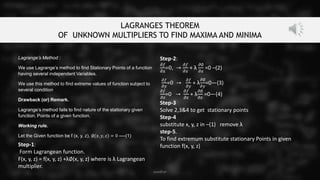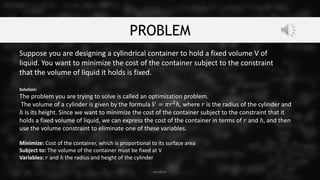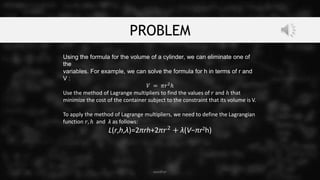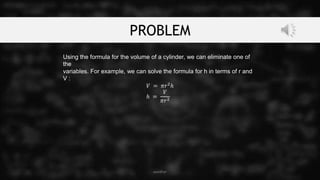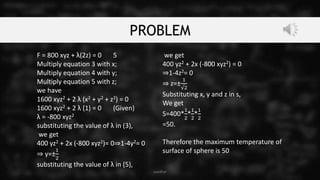1 de 19
Anúncio

### AEM.pptx

1. ADVANCED ENGINEERING MATHEMATICS Submitted by: Name : M.SAI SASIDHAR Regd No.: 221FA18091 Batch : 2 Sec. : 23(AIML) Submitted to: Dr.S.H.Manjula
2. MAXIMA AND MINIMA Maxima and minima are the peaks and valleys in the curve of a function. There can be any number of maxima and minima(local) for a function. In calculus, we can find the maximum and minimum value of any function without even looking at the graph of the function. Maxima will be the highest point on the curve within the given range and minima would be the lowest point on the curve. sasidhar
3. MAXIMA AND MINIMA OF TWO VARIABLES MAXIMA AND MINIMA OF TWO VARIABLES • Let f(x,y) be a function of two independent variables x and y, which is continuous for all values of x and y in the neighborhood of (a,b) i.e. (a+h,b+k) be a point in its neighborhood which lies inside the region R. • The point (a,b) is called a point of relative minimum, if f(a,b) < f(a+h,b+k) for all h,k Then f(a,b) is called the relative minimum value. • The point (a,b) is called a point of relative maximum, if f(a,b) > f(a+h,b+k) for all h,k Then f(a,b) is called the relative maximum value. sasidhar
4. MAXIMA AND MINIMA OF TWO VARIABLES • Stationary point: The point at which function is either maximum or minimum is known as stationary point. • Extreme Value: The value of the function at stationary point is known as extreme value of the function. • Saddle point : A point on the surface of the graph of a function where the slopes (derivatives) in orthogonal directions are all zero (a critical point), but which is not a local extremum of the function. sasidhar
5. MAXIMA AND MINIMA OF TWO VARIABLES Step 3: 1) If ln − 𝑚2 > 0 & l < 0 at (a,b), then f(x, y) is maximum at (a,b) & maximum value of the function is f (a,b). 2) If ln − 𝑚2>0 & l > 0 at (a,b), then f(x,y) is maximum at (a,b) & minimum value of the function is f (a,b). 3) If ln − 𝑚2< 0 at (a,b), then f (x,y) is neither maximum nor minimum at (a,b).Such point is called Saddle Point. 4)If ln − 𝑚2 < 0 at (a,b), then no conclusion can be made about the extreme values of f(x, y) & further investigation is required. sasidhar
6. APPLICATIONS OF MAXIMA AND MINIMA APPLICATIONS OF MAXIMA AND MINIMA IN DAILY LIFE Such applications exist in economics, business, and engineering. Many can be solved using the methods of differential calculus described above. For example, in any manufacturing business it is usually possible to express profit as a function of the number of units sold. sasidhar
7. PROBLEM Show that the function f(x , y) = x3 + y3 -63(x + y) +12xy is maximum at (-7 , -7) and minimum at (3 , 3). And also find maximum and minimum values ? Solution: Given, f(x , y) = x3 + y3 -63(x + y) +12xy Now from the given function 𝜕𝑓 𝜕𝑥 = 3x2 – 63 + 12y And 𝜕𝑓 𝜕𝑦 = 3y2 – 63 + 12x sasidhar
8. PROBLEM sasidhar Let ∂f/∂x = 0 (1) and ∂f/∂y = 0 (2) 3x2 – 63 + 12y = 0 3y2 – 63 + 12x = 0 We can also write it as, 3(x2 + 4y – 21) = 0 x2 + 4y – 21 = 0 (3) 3(y2 + 4x – 21) = 0 y2 + 4x – 21 = 0 (4) By solving the above two equations, we get x2 – y2 – 4(x – y) = 0 (x – y) (x + y – 4) = 0
9. PROBLEM x = y (5) and x + y = 4 (6) Now equation(5) in equation(3) then it becomes x2 + 4y – 21 = 0 sasidhar x2 + 7x – 3x – 21 = 0 x(x + 7) – 3(x + 7) = 0 (x – 3) (x + 7) = 0 x = 3 , -7 x values in equation (4) it becomes if x = -7 then y = -7 if x = 3 then y = 3 The two stationary points are (-7,-7) and (3,3)
10. PROBLEM We know that l = 𝜕2𝑓 𝜕𝑥2 = 6x m = 𝜕2𝑓 𝜕𝑥𝜕𝑦 = 12 n = 𝜕2𝑓 𝜕𝑦2 = 6y Now ln – m2 6x(6y) – (12)2 36xy – 144 At point (-7 , -7) l = 6x = 6(-7) = -42 sasidhar ln - m2 = 36xy – 144 = 36(-7)(-7) – 144 = 1764 – 144 = 1620 In this point l < 0 ; ln - m2 > 0 At point (3 , 3) l = 6x = 6(3) = 18 ln - m2 = 36xy – 144 = 36(3)(3) – 144 = 324 – 144 = 180 In this point l > 0 ; ln - m2 > 0
11. PROBLEM At point (-1 , 5) l = 6x = 6(-1) = -6 ln - m2 = 36xy – 144 = 36(-1)(5) – 144 = -180 – 144 = -324 In this point l < 0 ; ln - m2 < 0 And At point (5 , -1) l = 6x = 6(5) = 30 ln - m2 = 36xy – 144 = 36(5)(-1) – 144 = -180 – 144 = -324 In this point l > 0 ; ln - m2 < 0 f(x ,y) has maximum value at (-7 , -7) and minimum value at (3 , 3) Now to find the maximum and minimum value we substitute the points in given function f(x , y) = x3 + y3 -63(x + y) +12xy f(-7 , -7) = (-7)3 +(-7)3 – 63(-7-7) + 12(-7)(-7) = -343-343+882-588 = -392 The maximum value is -392 f(x , y) = x3 + y3 -63(x + y) +12xy f(3 , 3) = (3)3 + (3)3 – 63(3 + 3) + 12(3)(3) = 27 + 27 – 378 + 108 = -216 The minimum value is -216. sasidhar
12. LAGRANGES THEOREM OF UNKNOWN MULTIPLIERS TO FIND MAXIMA AND MINIMA sasidhar Lagrange’s Method : We use Lagrange’s method to find Stationary Points of a function having several independent Variables. We use this method to find extreme values of function subject to several condition Drawback (or) Remark. Lagrange’s method fails to find nature of the stationary given function. Points of a given function. Working rule. Let the Given function be f (x, y, z), ∅ 𝑥, 𝑦, 𝑧 = 0 ----(1) Step-1: Form Lagrangean function. F(x, y, z) = f(x, y, z) +λ∅(x, y, z) where is λ Lagrangean multiplier. Step-2: 𝜕𝑓 𝜕𝑥 =0, → 𝜕𝑓 𝜕𝑥 + λ 𝜕∅ 𝜕𝑥 =0 –(2) 𝜕𝑓 𝜕𝑦 =0 → 𝜕𝑓 𝜕𝑦 + λ 𝜕∅ 𝜕𝑦 =0—(3) 𝜕𝑓 𝜕𝑧 =0 → 𝜕𝑓 𝜕𝑧 + λ 𝜕∅ 𝜕𝑧 =0—(4) Step-3 Solve 2,3&4 to get stationary points Step-4 substitute x, y, z in –(1) remove λ step-5. To find extremum substitute stationary Points in given function f(x, y, z)
13. PROBLEM sasidhar Suppose you are designing a cylindrical container to hold a fixed volume V of liquid. You want to minimize the cost of the container subject to the constraint that the volume of liquid it holds is fixed. Solution: The problem you are trying to solve is called an optimization problem. The volume of a cylinder is given by the formula 𝑉 = 𝜋𝑟2ℎ, where 𝑟 is the radius of the cylinder and ℎ is its height. Since we want to minimize the cost of the container subject to the constraint that it holds a fixed volume of liquid, we can express the cost of the container in terms of 𝑟 and ℎ, and then use the volume constraint to eliminate one of these variables. Minimize: Cost of the container, which is proportional to its surface area Subject to: The volume of the container must be fixed at V Variables: 𝑟 and ℎ the radius and height of the cylinder
14. PROBLEM sasidhar Using the formula for the volume of a cylinder, we can eliminate one of the variables. For example, we can solve the formula for h in terms of r and V : 𝑉 = 𝜋𝑟2ℎ Use the method of Lagrange multipliers to find the values of 𝑟 and ℎ that minimize the cost of the container subject to the constraint that its volume is V. To apply the method of Lagrange multipliers, we need to define the Lagrangian function 𝑟, ℎ andλλ as follows: L(r,h,λ)=2πrh+2π𝑟2 + λ(V−πr2h)
15. PROBLEM sasidhar Using the formula for the volume of a cylinder, we can eliminate one of the variables. For example, we can solve the formula for h in terms of r and V : 𝑉 = 𝜋𝑟2 ℎ ℎ = 𝑉 𝜋𝑟2
16. PROBLEM sasidhar Using the formula for the volume of a cylinder, we can eliminate one of the variables. For example, we can solve the formula for h in terms of r and V : 𝑉 = 𝜋𝑟2 ℎ ℎ = 𝑉 𝜋𝑟2
17. PROBLEM sasidhar Using the formula for the volume of a cylinder, we can eliminate one of the variables. For example, we can solve the formula for h in terms of r and V : 𝑉 = 𝜋𝑟2 ℎ ℎ = 𝑉 𝜋𝑟2
18. PROBLEM sasidhar F= 400 xz2 + λ(2y) = 0 4 ∂s∂z+ λ ∂F∂z =0 F = 800 xyz + λ(2z) = 0 5 Multiply equation 3 with x; Multiply equation 4 with y; Multiply equation 5 with z; we have 1600 xyz2 + 2 λ (x2 + y2 + z2) = 0 1600 xyz2 + 2 λ (1) = 0 (Given) λ = -800 xyz2 substituting the value of λ in (3), we get 400 yz2 + 2x (-800 xyz2)= 0⇒1-4y2= 0 ⇒ y=± 1 2 substituting the value of λ in (5), we get 400 yz2 + 2x (-800 xyz2) = 0 ⇒1-4z2= 0 ⇒ z=± 1 √2 Substituting x, y and z in s, We get S=400* 1 2 * 1 2 * 1 2 =50. Therefore the maximum temperature of surface of sphere is 50
19. THANK YOU sasidhar
Anúncio